Mathematical and Physical Journal
for High Schools
Issued by the MATFUND Foundation
 Already signed up? New to KöMaL?

# KöMaL Problems in Informatics, May 2015

Show/hide problems of signs:## Problems with sign 'I'

Deadline expired on June 10, 2015.

I. 376. Crossword puzzles are popular games and have many variants. In its usual form, words are separated by black squares. When words are entered correctly, solutions can be read horizontally from left to right and vertically from top to bottom. The numbering of the squares begins in the top left corner where the first horizontal or vertical solution word should start, then each square in the puzzle is numbered where a horizontal or vertical word starts. The puzzles in our task do not contain words with one letter. If a square contains the first letter of a word that is simultaneously a horizontal and a vertical solution, then the square gets only one number.

The example shows an empty crossword and its numbered counterpart.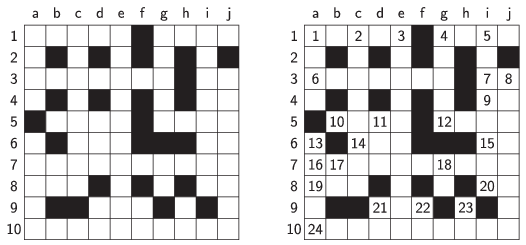To test your solution, the halo.txt file can be downloaded from our web site, containing the description of a crossword of size $\displaystyle N\times M$ ($\displaystyle 5\le N, M\le 15$). The first line of the file contains the values of $\displaystyle N$ and $\displaystyle M$ separated by a space. The following $\displaystyle N$ lines describe the squares: the characters f'' and ''.'' represent the black and the empty squares, respectively.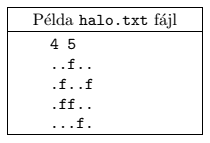If an output is displayed on the screen, the number of the actual task should also appear. Whenever the program prompts the user to enter some data, the type of data should also be displayed; for example, in the 4th task: Task #4 - Please enter the coordinates of a square: ''. Diacritical marks in the output can be omitted.

1. By using the data read from the file halo.txt, solve the following tasks.

2. Display on the screen the number of black and the number of empty squares in the puzzle.

3. Determine which row contains the most black squares. If there is more than one such row, the one with the smallest row number should be displayed. Then repeat this counting for the columns.

4. Prompt the user to enter the coordinates of a square in the crossword (e.g. 10h), then display whether it will get a number or not.

5. Display on the screen the number of words with letters $\displaystyle 2, 3,\dots, 10$ that can be placed horizontally in the puzzle.

6. Number the squares in the puzzle according to the rules, then write the result into the file szamozott.txt. The content of each square should occupy 3 characters (see the Példa'' example below).

7. Display the number of definitions corresponding to the horizontal and to the vertical words.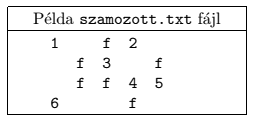The source code (i376.pas, i376.cpp, ...) of your program with a short documentation (i376.txt, i376.pdf, ...) of your program and solution, also describing which developer environment to use for compiling the source, should be submitted.

(10 pont)

solution, statistics

I. 377. The site oktatas.hu publishes the final results of the OKTV contests in each year:

http://www.oktatas.hu/kozneveles/tanulmanyi_versenyek/oktv_kereteben/dijazottak_eredmenyek

Results for each subject are available as PDF documents. Although one can perform simple text search in this format, but advanced search or statistical processing of the data is not supported.

Your task is to design a database to store the contest results, as well as the process to transfer data from the files to the database. To test your work, you should load the data corresponding to the last 5 years of the applied information technology contest into your database.

You can use only the files available at the web address given above. The necessary conversion steps may be performed by using any (if possible, freely available) program.

Since end-users use the default file format of Microsoft Access, or MySQL dump files, your database should have any of these formats.

The description of your solution (i377.pdf) and your database (i377.accdb, i377.sql) should be submitted in a compressed file (i377.zip). Your description should contain an image modeling the database (i.e. the connections between the tables), the name and version number of the database application, your reproducible method to process the PDF files, and any technical or content-related issues you encountered together with their solutions.

(10 pont)

solution, statistics

I. 378. We are given a black-and-white image of $\displaystyle N\times M$ pixels, described by 0s and 1s arranged in a grid. An image is considered to be nicer, if there are more adjacent pixels that are identical. In this exercise, two pixels are adjacent if they share a common edge. Your goal is to make the original image nicer by negating certain pixel values. Negating the value of a single pixel costs $\displaystyle Q$ units of money. However, in the final image, every pair of adjacent pixels with different colors will result in an additional penalty of $\displaystyle P$ units of money.

For some given images you should find the transformation such that the sum of the $\displaystyle P$ and $\displaystyle Q$ quantities is the smallest.

Without submitting a program, your task now is the convert the three input files in.1, in.2 and in.3 (downloadable from our web site) to out.1, out.2 and out.3. The first line of an input file contains 4 integers ($\displaystyle N$, $\displaystyle M$, $\displaystyle P$ and $\displaystyle Q$), describing the number of rows and columns of the grid, and the cost and penalty parameters. The image itself is described in the following $\displaystyle N$ lines, each containing $\displaystyle M$ characters. The output should have a similar format with an $\displaystyle N\times M$ grid of pixel values. You are not required to submit an optimal solution, but solutions will compete against one another: the solution with the smallest total $\displaystyle P+Q$ value for the 3 images will obtain 10 points, and other, suboptimal solutions will get proportionally less points.

A sample input file is presented below, together with a possible (not necessarily optimal) transformation. The total cost $\displaystyle P+Q$ here is $\displaystyle 6\cdot 2+3\cdot 3 =21$ units of money.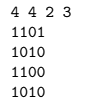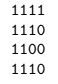The three output files should be submitted in a compressed file (i378.zip).

(10 pont)

solution, statistics## Problems with sign 'S'

Deadline expired on June 17, 2015.

S. 99. We notice that $\displaystyle N$ cracks ($\displaystyle 1\le N\le 5000$) appeared on a wall of length $\displaystyle M$ ($\displaystyle 1\le M\le 100\;000$, with integer $\displaystyle M$). In this exercise, cracks are modeled as points, and their location is given by some integer coordinates $\displaystyle x$ ($\displaystyle 1\le x\le M$) measured from one end of the wall. Our goal is to cover all cracks on the wall with paint.

By opening a bucket of paint labeled $\displaystyle w$'', we can paint a wall segment of length exactly $\displaystyle w$, bounded by the coordinates $\displaystyle x_0$ and $\displaystyle x_1$ ($\displaystyle w=x_1-x_0+1$). Within this wall segment we can of course cover the intact parts of the wall as well, even multiple times. We keep on opening the buckets and painting until all cracks are covered. For each integer $\displaystyle w$ ($\displaystyle 1\le w\le M$), we have one bucket of paint labeled $\displaystyle w$'' and with known cost $\displaystyle b_j$. Painting a longer wall segment may cost less than painting a shorter one; and it may also happen that the price of a bigger bucket is less than or equal to the price of a smaller bucket. Your task is to cover all cracks on the wall for the minimum cost.

Your program should read the values of $\displaystyle N$ and $\displaystyle M$ from the first line of the standard input, then the $\displaystyle a_i$ integers (describing the crack locations) from the following $\displaystyle N$ lines. The next $\displaystyle M$ lines contain the paint bucket prices $\displaystyle b_j$ corresponding to the consecutive lengths. The first line of the standard output should contain the minimum cost necessary to complete the wall painting. To save space, instead of displaying numbers in $\displaystyle N$ and $\displaystyle M$ input lines, they appear in one line and are separated with / characters.

In the example, Példa bemenet'' is a sample input, while Példa kimenet'' is the corresponding output. In this situation, 3 paint buckets with labels 4'', 1'' and 2'' are sufficient to paint all the cracks; the cost is $\displaystyle 4+2+3=9$.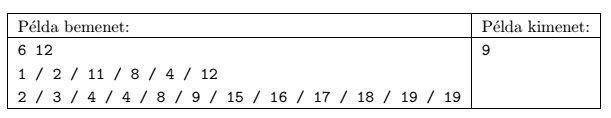Scoring and bounds. You can obtain 1 point for a brief and proper documentation clearly describing your solution. Nine further points can be obtained provided that your program solves any arbitrary valid input within 1 second of running time.

The source code (s99.pas, s99.cpp, ...) without the .exe or any other auxiliary files generated by the compiler but with a short documentation (s99.txt, s99.pdf, ...), also describing which developer environment to use for compiling your source, should be submitted in a compressed file s99.zip.

(10 pont)

solution, statistics

\$Var(Body)